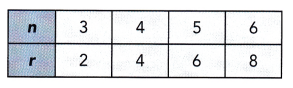# Math in Focus Grade 8 Chapter 3 Lesson 3.4 Answer Key Solving for a Variable in a Two-Variable Linear Equation

Go through the Math in Focus Grade 8 Workbook Answer Key Chapter 3 Lesson 3.4 Solving for a Variable in a Two-Variable Linear Equation to finish your assignments.

## Math in Focus Grade 7 Course 3 A Chapter 3 Lesson 3.4 Answer Key Solving for a Variable in a Two-Variable Linear Equation

### Math in Focus Grade 8 Chapter 3 Lesson 3.4 Guided Practice Answer Key

Question 1.
Express x in terms of y for the equation 2(x – 3) =3y -1. Find the value of x when y = 3.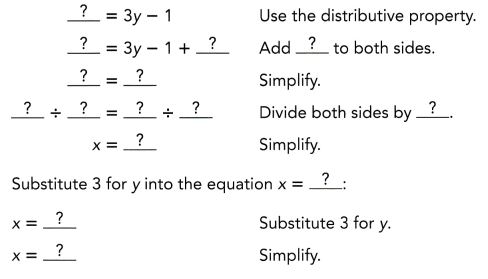x = 7,

Explanation: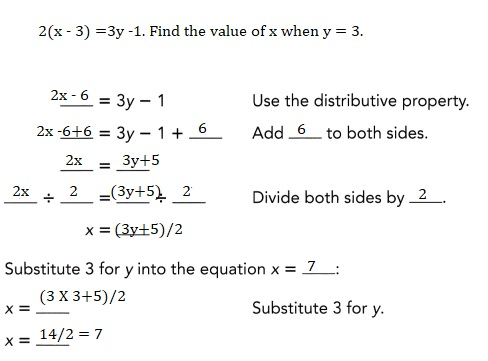Question 2.
Express x in terms of y for the equation y = $$\frac{2 x+3}{2}$$. Find the value of x when y = -13.
x = -29/2,

Explanation: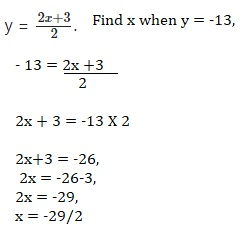Question 3.
The mean (or average) of three numbers x, x$$\sqrt{3}$$ and 2 is M.

a) Express x in terms of M.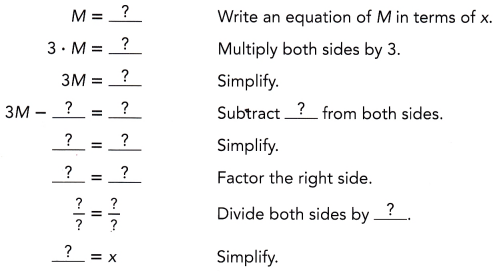x = 3M-2/2.732
Explanation: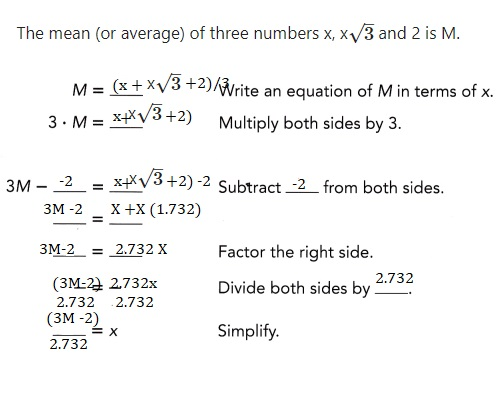b) Create a table of values for M and x when M = 0, 1, 2, and 3.
Round each x-value to the nearest hundredth.
Substitute 0, 1, 2, and 3 for M into the equation x =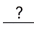.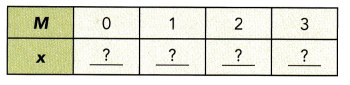When M = 0, X = -0.732,
M= 1, x =0.366, M=2 , x = 1.464 and M = 3 , x= 2.562,
Explanation: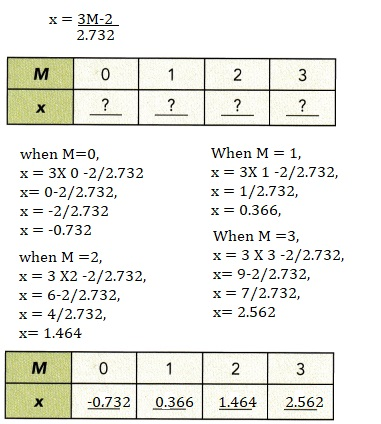### Math in Focus Course 3A Practice 3.4 Answer Key

Express y in terms of x. Find the value of y when x = -1.

Question 1.
5 – y = 3x
y = 5 – 3x,y = 8

Explanation:
Given 5 -y = 3x, x=-1,
Expressing y in terms of x as y = 5-3x and as x = -1
y = 5 – 3(-1)= 5 +3 = 8.

Question 2.
-3(x + 2) = 5y
y =-3/5(x+2), y = -3/5,

Explanation:
Given -3(x+2) = 5y and x = -1
Expressing y in terms of x as y = -3/5(x +2),
y = -3/5(-1+2),
y= -3/5(1),
y = -3/5.

Question 3.
6(x – y) = 19
y= x -19/6, y = -25/6,

Explanation:
Given 6(x-y)=19,
6x – 19 =  6y,
y = x – 19/6,when x = -1,
y = -1 -19/6 = -25/6.

Question 4.
4x – 3 = 0.4x – 2y
y= 1.5 – 1.8 x, y = 3.3,

Explanation:
Given 4x – 3 =0.4x -2y,
4x – 3-0.4x = -2y
3.6x -3 =-2y
y = -3.6x/2 + 3/2
y = 1.5 – 1.8x, when x = -1
y = 1.5 -1.8(-1)= 1.5+1.8 = 3.3.

Question 5.
$$\frac{1}{6}$$x + $$\frac{3}{4}$$y = 4
y = (96-4x)/18,y = 5.5

Explanation:
Given$$\frac{1}{6}$$x + $$\frac{3}{4}$$y = 4 multiplying both sides with 24,
4x +18 y =96,
18 y = 96-4x
y = (96- 4x)/18,when x = -1,
y = (96-4(-1))/18 = 96+4/18 = 100/18 = 5.5.

Question 6.
0.5y – 2 = 0.25x
y = 4+0.5x, y = 3.5,

Explanation:
Given 0.5y – 2 = 0.25x,
0.5 y = 2 +0.25x
y = 4 +0.5 x, when x = -1,
y = 4 +0.5(-1) = 4 -0.5 = 3.5.

Express x in terms of y. Find the value of x when y = 5.

Question 7.
5x – y = 3(x + y)
x = 2y, x = 10,

Explanation:
Given 5x – y = 3(x+y),
5x – y = 3x +3y,
5x -3x = 3y+y,
2x = 4y,
x = 2y,when y = 5,
x = 2 X 5 = 10.

Question 8.
3(x + 2y) = 2x + 5y
x = -y, x = -5,

Explanation:
Given 3(x+2y)=2x +5y,
3x +6y = 2x +5y,
3x – 2x = 5y-6y,
x = -y,when y =5, x = -5.

Question 9.
1.5(x – y) = 1
x= 0.66 +y, x = 5.66,

Explanation:
Given 1.5(x-y)=1,
1.5x = 1+ 1.5 y,
x = 0.66 + y,when y = 5, x = 0.66 + 5,
x = 5.66.

Question 10.

2y + 8 = $$\frac{1}{4} x$$
x =(8y+32), x = 72,

Explanation:
Given 2y+ 8 = $$\frac{1}{4} x$$,
4(2y+8)= x,
x = 8y + 32, when y = 5,
x = 8 X 5+ 32= 40 +32 = 72.

Question 11.
$$\frac{2(x-3)}{y}$$ = 5
x = (5y+6)/2, x = 31/2,

Explanation:
Given $$\frac{2(x-3)}{y}$$ = 5,
2(x -3)=5y,
2x – 6 = 5y,
2x = 5y +6,
x =(5y +6)/2,when y = 5,
x = (5 X 5 +6)/2,
x = 31/2.

Question 12.
$$\frac{1}{3}(6 x-1)$$ = $$\frac{6 y}{5}$$
x= (18y+5)/30, x =95/30,

Explanation:
Given$$\frac{1}{3}(6 x-1)$$ = $$\frac{6 y}{5}$$,
5(6x-1) = 18y,
30 x- 5 = 18y,
30x = 18y +5,
x=(18y+ 5 )/30, when y =5,
x = (18X 5+5)/30= 95/30.

Question 13.
The perimeter, P inches, of a semicircle of diameter, d inches, is represented by P = 0.5πd+ d.

a) Express d in terms of P.
p = d(0.5π +1) inches,

Explanation:
Given the perimeter, P inches, of a semicircle of diameter,
d inches, is represented by P = 0.5πd+ d so p= d(0.5π +1) inches.

b) Find the diameter if the perimeter is 36 inches.
Use $$\frac{22}{7}$$ as an approximation for π.
The diameter is 14.007 inches,

Explanation:
Given to find the diameter if the perimeter is 36 inches.
Using $$\frac{22}{7}$$ as an approximation for π= 3.14,
we have p = d(0.5π +1) inches,
36 = d(0.5 X 3.14 +1),
36 = d(1.57 +1),
36 = d (2.57),
d = 36/2.57= 14.007 inches.

Question 14.
The horizontal distance, X inches, and vertical distance,
Y inches, of each step of a staircase are related by the linear equation
X = $$\frac{1}{2}$$(20 + Y).

a) Express Y in terms of X.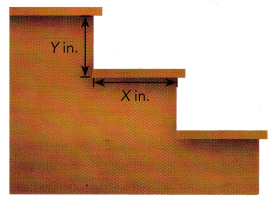Y = 2X – 20,

Explanation:
Given X = $$\frac{1}{2}$$(20 + Y),
2 X = 20 + Y,
Y = 2X – 20.

b) Complete the table below.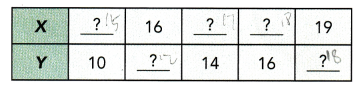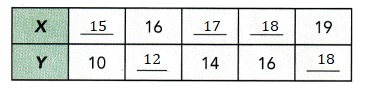Explanation:
We have Y = 2X – 20,
when Y = 10, 2X = Y + 20 = 2X = 30, X =15,
When X = 16, Y = (2x-20) = 2X 16 – 20 = 32 – 20 =12,
When Y = 14, 2X = Y +20 ,2 X = 14 + 20 = 34 , X =17,
When Y =16, 2X = 16+20 = 36, X = 36/2 = 18,
When X = 19,Y =(2x -20)= 2X 19 – 20 = 38 – 20 = 18,
Completed the table above.

c) Find the value of X and Y if X = Y.
If X = Y then Y = 20,

Explanation:
We have Y =2X – 20,
Y = 2 Y -20,
2Y -Y =20,
Y = 20.

Question 15.
Use the isosceles triangle.

a) Write an equation for y in terms of x.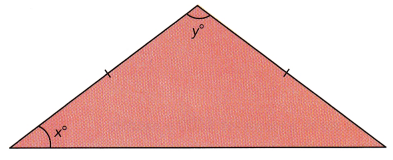x + y = 90,

Explanation:
Given the triangle is isosceles is 2(x +y)+ 90 = 180,
2(x+y) = 180-90= 90,
x + y = 90/2 = 45.

b) Find the value of y when x = 24.5.
y = 65.5,

Explanation:
We have x +y = 90, when x = 24.5 ,
y = 90-24.5 = 65.5.

Question 16.
At a travel agency, the cost of a trip to Mexico is $350 for an adult and$200 for a child.
One month, the agency sold 50 trips. Of these trips, y trips were for children.
The travel agency collected C dollars from selling all 50 trips.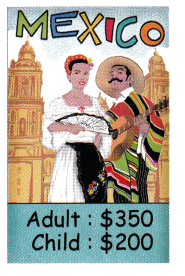a) Write a linear equation for C in terms of y.
C =350(50 -y)+ 200 y,

Explanation:
Given at a travel agency, the cost of a trip to Mexico is $350 for an adult and$200 for a child.
One month, the agency sold 50 trips. Of these trips, y trips were for children.
The travel agency collected C dollars from selling all 50 trips. is
C =350(50 -y)+ 200 y.

b) Express y in terms of C.
y = (17,500 – C)/150,

Explanation:
We have C =350(50 -y)+ 200 y,
C = 17,500 – 350y + 200y,
C = 17,500 – 150y,
150 y = 17,500 -C
y = (17,500 – C)/150.

c) If $15,250 was collected, find the number of children going on the trip. Answer: The number of children going on the trip is 15, Explanation: If$15,250 is collected the number of children going on the trip,
we have y= (17,500-c)/150,
y= (17,500-15,250)/150,
y= 2,250/150= 15.

Question 17.
A trash disposal company charges accordinq to the weight of trash it disposes.
The charge, y dollars, for x pounds of trash is represented by the equation
y = 2($$\frac{1}{4}$$x – 10).

a) Write an equation for x in terms of y.
x = 2x +40,

Explanation:
y = 2($$\frac{1}{4}$$x – 10),
y = 1/2 x – 20,
1/2 x = y +20,
x = 2y +40.

b) Create a table of x- and y-values for y = 60, 80, 100, 120, and 140.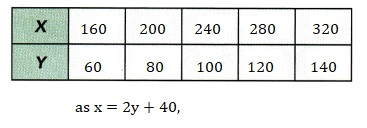Explanation:
We have x = 2y + 40,
when y =60, x = 2X 60+ 40 =120 + 40 = 160,
when y = 80, x = 2 X 80 + 40 = 160 + 40 = 200,
when y = 100, x = 2 X 100+ 40 = 200 + 40 = 240,
when y = 120 , x = 2 X 120 + 40 = 240 + 40 = 280,
when y = 140, x = 2 X 140 + 40 = 280 + 40 = 320,
Created a table of x and y values above.

Question 18.
The mean, or average, of three numbers 17.4, 23.8, and x is M.

a) Write an equation for x in terms of M.
x = 3M – 41.2,

Explanation:
Given the mean or average of three numbers 17.4, 23.8, and x is M so
M = (17.4 + 23.8 +x)/3,
3 M = x + 41.2,
x = 3M – 41.2.

b) Create a table of values for M = 15, 17, 19, 21, and 23.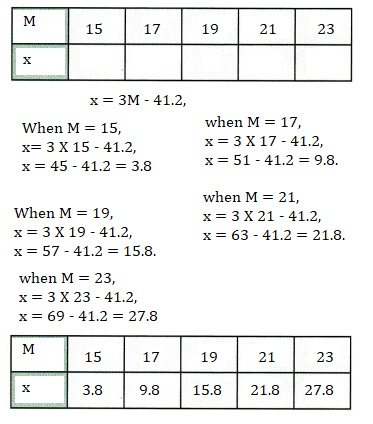Explanation:
Created the tables of values M = 15,17,19,21 and 23,
so x = 3.8,9.8,15.8,21.8,27.8 respectively.

Question 19.
A rectangle has a width of w units and a length of 5 units.
Its perimeter is given by P = 2(w + 5). Solve for w in terms of P.
Create a table of values for P = 12, 14, 16, and 18.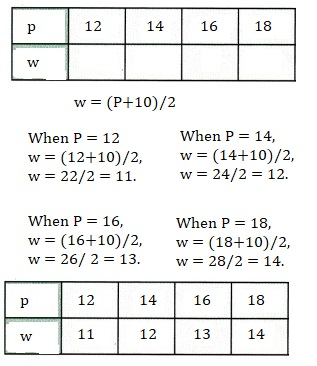Explanation:
Given P = 2(w+5),
2w +10 = P,
2w = P + 10,
w = (p+10)/2,
Craeted a table of values for P=12,14,16 and 18 as shown above.

Question 20.
In the number pattern 3, 7, 11, 15, 19, …, each new number is
4 greater than the previous number. To find the number L in the nth position,
use the formula L = 3 + 4(n – 1).

a) What number is in the tenth position?
39 is in the tenth position,

Explanation:
Given the number pattern 3, 7, 11, 15, 19, …, each new number is
4 greater than the previous number. To find the number L in the nth position,
we use the formula L = 3 + 4(n – 1) when n = tenth position,
L = 3 +4(10-1),
L = 3 + 4(9),
L = 3 + 36= 39.

b) In which position is the number 63?
In 16th position is the number 63.

Explanation:
We have L= 3+4(n -1)  where L is the number and n will be position
if the number is 63 the position is 63 = 3+4(n-1),
63 -3 = 4(n-1),
60 = 4(n-1),
n -1 = 60/4,
n -1 = 15,
n = 15+1 = 16.

Brain @ Work

Question 1.
Lynnette runs a private tutoring business. She rents a room for $500 a month, which is her only expense. She charges$50 an hour per student, and gives each student two lessons per month. Each lesson lasts 1.5 hours.

a) Write an equation for her monthly profit, P, in terms of s, the number of students she has.
P = $50s –$500,

Explanation:
Given Lynnette runs a private tutoring business.
She rents a room for $500 a month, which is her only expense. She charges$50 an hour per student, equation for her monthly profit P
in terms of s, the number of students she has is P = $50s –$500.

b) Find her monthly profit if she has 40 students.
If she has 40 students her montly profit is $1,500, Explanation: We have P =$50s – $500, if Lynnette has 40 students P =$50 X 40 – $500, P =$2,000 – $500 =$1500.

c) Find the minimum number of students she needs if she wants to make a monthly profit of at least $4,600.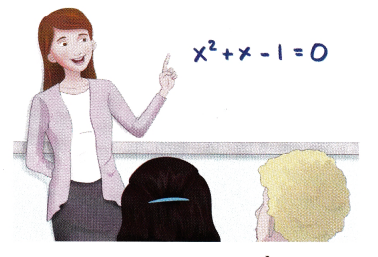Answer: 102 minimum number of students she needs if she wants to make a monthly profit of at least$4,600,

Explanation:
We have P = $50s –$500 if Lynnette wants to make a monthly profit of at least $4,600, the number of students s is$4,600 = $50s –$500,
$50s =$4,600+ $500,$50s = $5,100, s =$5,100/50 = 102.

Question 2.
Stefanie’s train will leave her train station in 24 minutes and
she is y miles from the station. To catch the train, she walks at a speed of
4 miles per hour and later runs at a speed of 8 miles per hour.
a) Write an equation in terms of y for the distance she has to walk, w,
to reach the station in 24 minutes.
w = (16-5y)/5 or  (3.2-y)

Explanation:
Given Stefanie’s train will leave her train station in 24 minutes and
she is y miles from the station. To catch the train, she walks at a speed of
4 miles per hour and later runs at a speed of 8 miles per hour.
Walk is w/4 and run (y-w)/8,
so w/4+(y -w)/ 8 = 24/60,
2w+y -w = 24 X 8/60,
w + y = 16/5,
w = (16-5y)/5 or 3.2 -y,

b) Solve for y in terms of w. How far is she from the station if she has to
walk 1 mile to reach the station on time?
y =2.2,

Explanation:
As we have w = (16-5y)/5 or 3.2 -y if she is from the station if she has to
walk 1 mile to reach the station on time then y = 3.2 – 1= 2.2.

c) Why do the values of y have to be between 1.6 and 3.2?
As walk cannot not zero or less than 4 miles,

Explanation:
If y values are not to be between 1.6 and 3.2 As walk cannot not zero or less than
4 miles if y = 3.2 then w = 3.2 – 3.2 = 0.

Question 3.
A polygon has n sides. The sum of the measures of a polygon’s
interior angles is equal to the sum of the measures of r right angles.
A table of r- and n-values is shown below.
Explain how you would find a linear equation involving r and n.